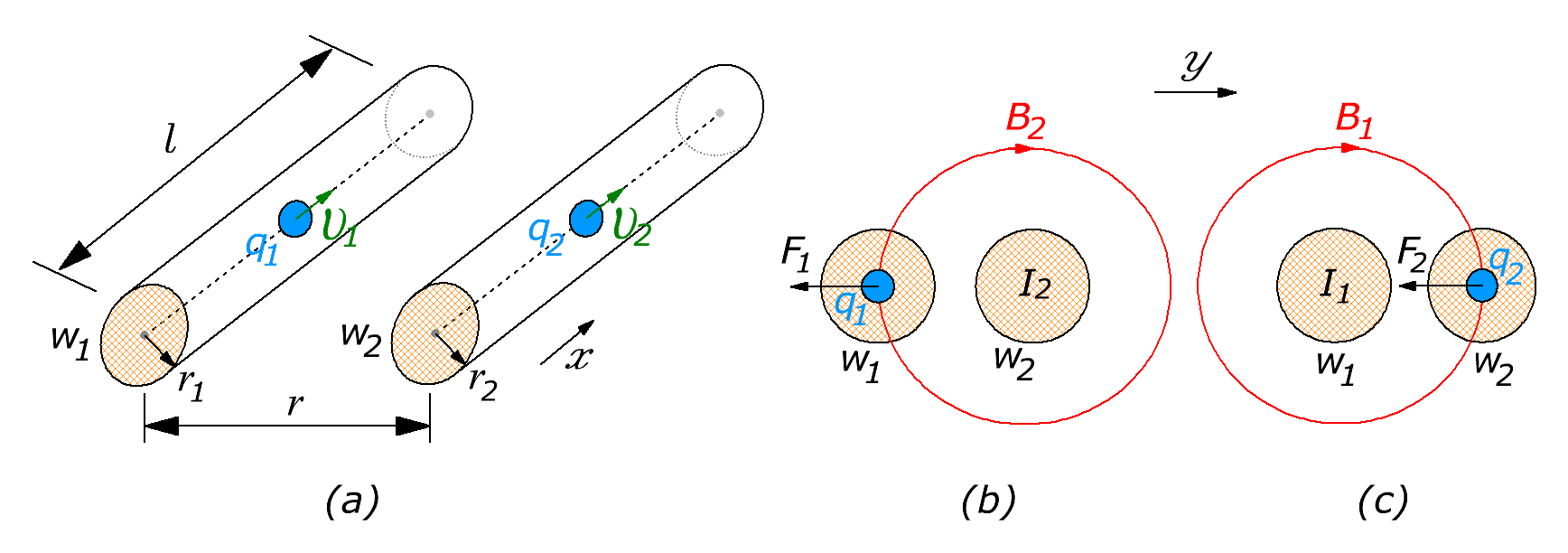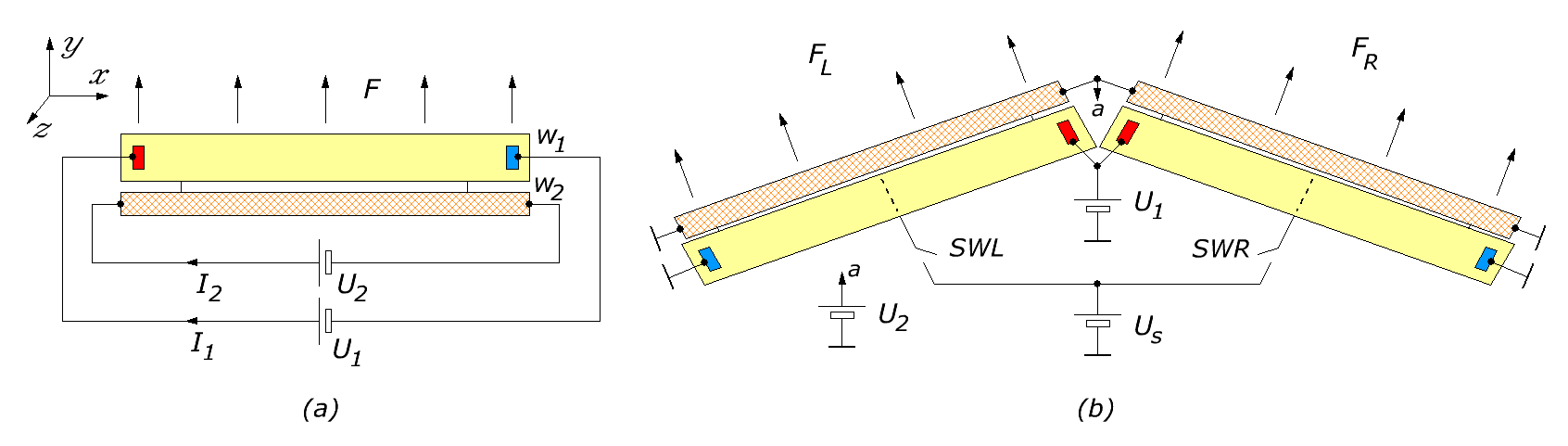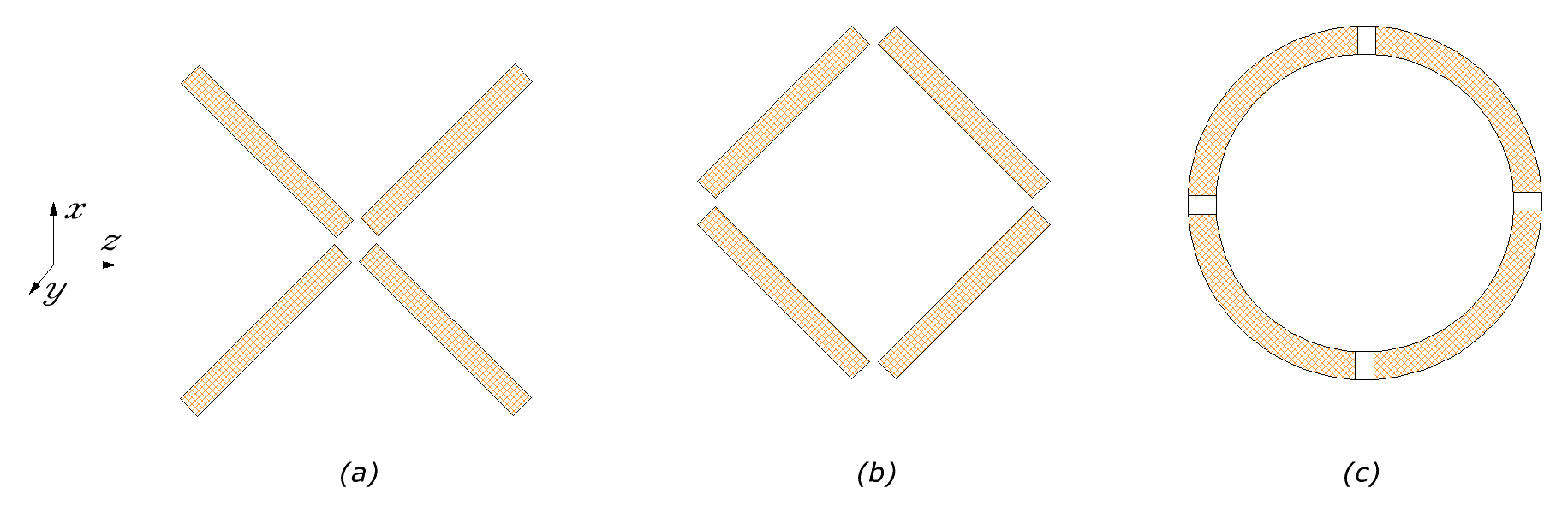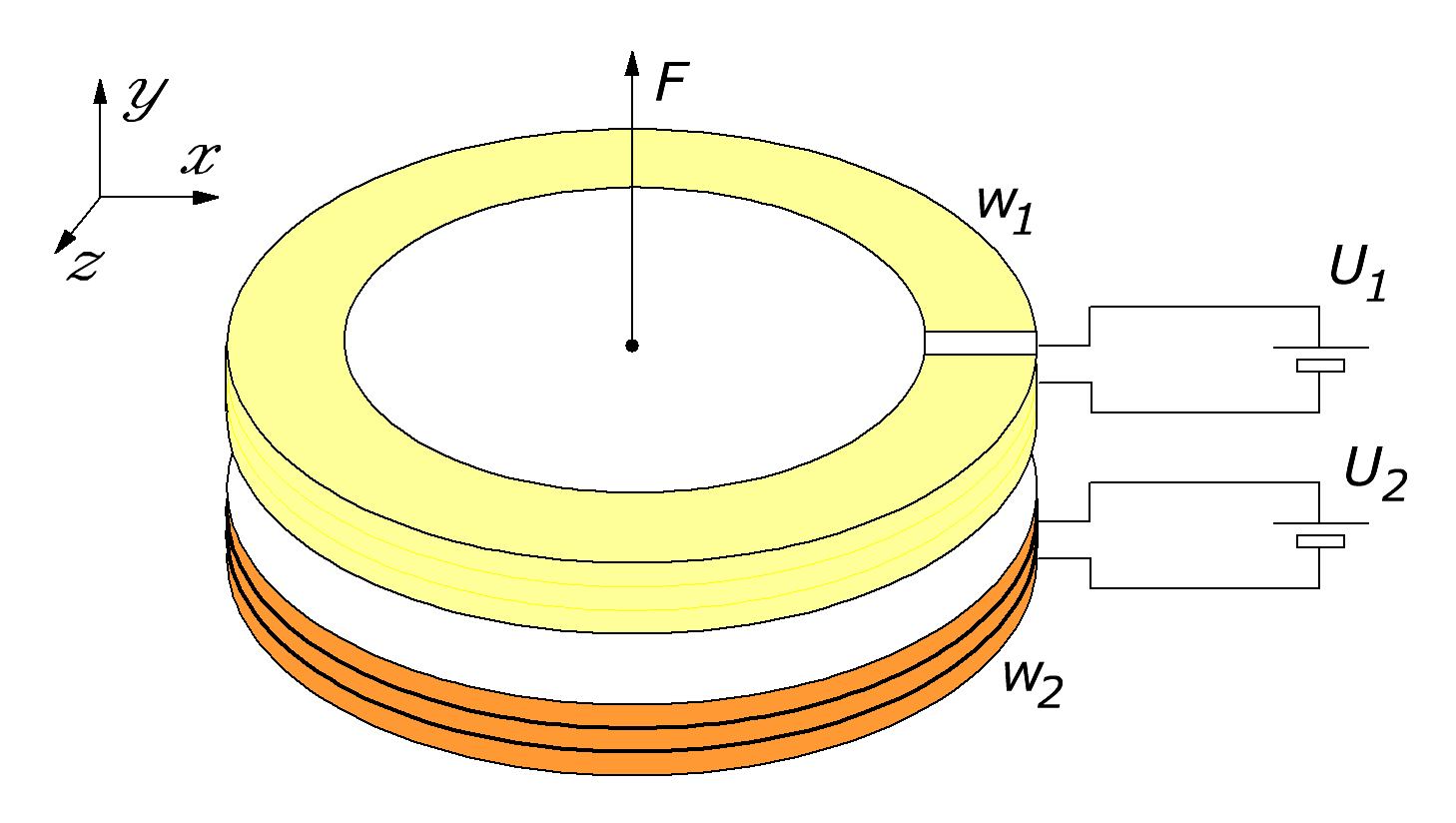Research website of Vyacheslav Gorchilin
2019-04-11
Unsupported mover on uncompensated charge
Cosmonautics Day I want to share an unusual idea, unsupported mover. It is based on the use of the Lorentz force  for two conductors, but with different speeds of the charges on them. Due to the difference of velocities between the charges arises uncompensated force representing the thrust of the propeller. This note will consist of mathematical and practical part, and will be devoted to its magnetic variant.
The mathematics of propulsion. Magnetic principle
Consider two conductors w1 and w2, each of which, along the axis of $$x$$, moves a charge $$q$$ with rate $$\vartheta$$ (Fig. 1a). The length of conductors is the same and equal to $$l$$, and the distance between their axes — $$r$$. In this situation, between the conductors arises ampere force , but since the conductors are interconnected mechanically, this force will be compensated. Another force will arise due to the different velocity of the charges and it will be uncompensated. It is what we are on and we will discuss it.Fig.1. The Lorentz force in two conductors with different speeds of the charges
The difference between conductors w1 and w2 is that the velocity of the charges in them are different: $\Delta\vartheta = \vartheta_1 - \vartheta_2 \qquad (1)$ Then, due to the difference of velocities and the movement of the power lines relative to the charges along the axis $$y$$ has additional strength (Fig. 1b, 1c): $F_1 = q_1 B_2 \Delta\vartheta, \quad F_2 = q_2 B_1 \Delta\vartheta \qquad (2)$ to find out what the charge moves in a conductor, you need to remember the formula for the current $$I=\Bbb{d}q / \Bbb{d}t$$, in which we take an infinitely small changes of the charge at time $$t$$. Hence we derive: $\Bbb{d}q_1 = I_1 \Bbb{d}t, \quad \Bbb{d}q_2 = I_2 \Bbb{d}t \qquad (3)$ Next, we find the total force $$F$$ and substitute there the previously obtained expression: $F = F_1 + F_2, \quad \Bbb{d}F = (B_2 I_1 + I_2 B_1) \Delta\vartheta\, \Bbb{d}t \qquad (4)$ If the direction of the currents are opposite, the total force will be, as the difference of its components. In integral form it will be so: $F = \int (B_2 I_1 + I_2 B_1) \Delta\vartheta\, \Bbb{d}t \qquad (5)$ But we are interested in the option when the difference between the velocities of the charges in the conductors is very large. In this case, the formula (5) can be converted so that the time and speed it will be replaced with the spatial coordinate. For this purpose there is sufficient to substitute the expression $$\Delta\vartheta \approx \vartheta_1 = \Bbb{d}x / \Bbb{d}t$$: $F = \int \limits_{0}^{l} (B_2 I_1 + I_2 B_1)\, \Bbb{d}x, \quad \vartheta_1 \gg \vartheta_2 \qquad (6)$ In all formulae below we assume the condition (6), which implies, that speed is $$\vartheta_1$$ is much larger $$\vartheta_2$$, and represents the actual velocity of charges. If you also assume approximate the ideal conditions in which the entire length of the conductors currents and magnetic fields are equal, then this formula can be recorded without the integral: $F = l\, (B_2 I_1 + I_2 B_1) \qquad (7)$ Continuing to idealize our model, we take the formula for an infinite conductor to calculate the magnetic field at the distant point from it: $B_1 = {\mu\mu_0 I_1 \over 2\pi r}, \quad B_2 = {\mu\mu_0 I_2 \over 2\pi r} \qquad (8)$ where: $$\mu_0$$ is the absolute magnetic permeability. In this case we assume that the distance $$r$$ is greater than the diameter of the conductor. If we believe that the relative magnetic permeability $$\mu$$ equal and uniform throughout the volume, the formula (7) turns into this: $F = l {\mu\mu_0 \over \pi } {I_1 I_2 \over r} \qquad (9)$ As seen from formula (9), we came to the ampere law  for the two current-carrying conductors, but with a qualitative difference: the speed of movement of charges in conductors are totally different. Hence uncompensated force $$F$$.
The practical part
One of the simplest options of propulsion, implemented on this principle, shown in (2a). There w1 is a vacuum tube with two electrodes, between which flows the current $$I_1$$. In this case, maybe the cathode will have to warm up for best release of electrons. As w2 is the conductor carrying a current $$I_2$$.
Power supplies can be constant voltage. Much less cost of creating the traction you can get, if you make the sources U1 and U2 with variable voltage and so that the currents were obtained, where possible, reactive. Of course, in this case, you will need to synchronize these sources in phase and frequency.Fig.2. Options for magnetic propulsion
Because in a vacuum tube, the charge will move with acceleration, there will be a bias force along its length. Therefore, the best solution would be to place two symmetrical clusters (Fig. 2b). In addition, in this case, you can control left and right thrust, including switches SWR and SWL , respectively. To control the currents in the tube is possible by means of a control grid, feeding her through those keys the bias voltage Us. When applying a reverse voltage on w2 thrust will occur in the opposite direction, which makes this mover makinerni in all directions in one plane. To maneuver in 3D will need another pair of symmetric clusters that are located in the perpendicular plane. Such incorporation can be called a classic "star" (Fig. 3a).Fig.3. Different inclusion clusters mover
Another interesting solution could be the inclusion of clusters "triangle", "diamond" (Fig. 3b) or its variation — "circle" (Fig. 3c). Each of these options mover allows you to maneuver in all planes, and has its advantages and disadvantages.Fig.4. Cell magnetic propulsion
If we talk about cellular structure mover, then a good option may be the cell shown in (4). Here w2 is a coil of metal wire, and w1 — vacuum lamp. Last, to achieve large instantaneous currents may be pulsed and controlled, and if the current in w2 is variable, the control lamp must be synchronized with one of his half-cycles. Even better and powerful result will be a simultaneous impulse pump as lamps w1and the conductor w2.

The materials used
1. Wikipedia. The Lorentz Force.
2. Wikipedia. The law of ampere.